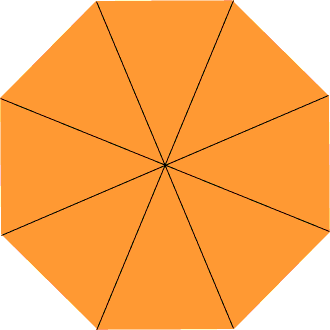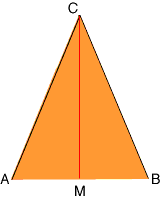Quandaries and Queries Who is asking: Parent Level: Middle Question: I am trying to determine what the size of each triangle is of a 12 foot circle consisting of 8 triangles would be. I've gotten as far as the radius being 6 feet, but cannot figure the distance. Hi, The shape you are describing is called a regular octagon.I drew in the diagonals to show the eight triangles. Here is one of them which I labeled CAB. I also drew a line from C to the midpoint M of AB.As you noted |CA| = |BC| = 6 feet. The angle BCA is one eighth of 360o so angle BCA is 45o. The angles CAB abd CBA have the same angle measurement since the triangle CAB is isosceles. The three angles in a triangle add to 180o angle CAB has angle measurement (180o - 45o)/2 = 67.5o. To completely describe the triangle all that remains is to find the length of the side AB. For this I need to use trigonometry. The cosine of the angle CAM is |AM|/|CA| and hence |AM| = |CA|cos(CAM) = 6cos(67.5o) = 60.3827 = 2.296 feet Since AM is half of AB, |AB| = 22.296 = 4.59 feet, which is approximately 4 feet 7 1/8 inches. Penny Go to Math Central